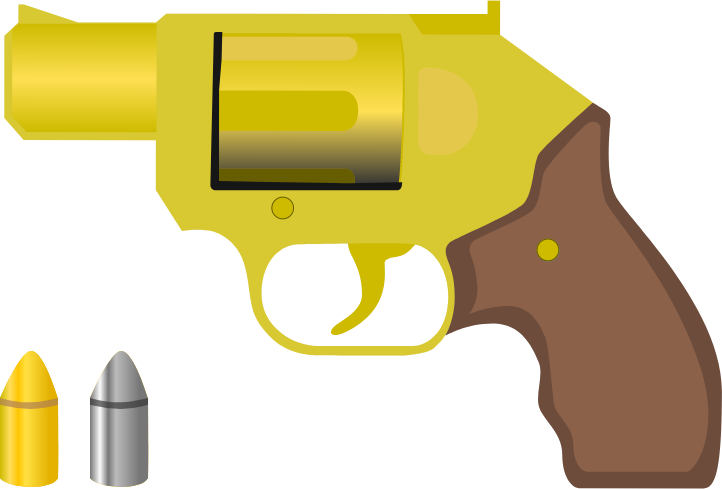# Problems of the Week

Contribute a problem

# 2018-01-01 Intermediate

Each of the digits from 0 to 9 appears in the number $2^{29}$ once except for one. Which digit is missing?


Hint: Divisibility RulesHow effective are gold bullets compared to lead bullets of the same shape and size?

Assume that the bullets do not change shape and that the bullets leave the gun at the same velocity.

If $x=0,$ then $x - x^2 + x^3 - x^4 + \cdots = 0.$

Which of the following is the value of $y - y^2 + y^3 - y^4 + \cdots ,$ where $y$ is some real number?

A Pythagorean triple is a set of positive integers $a < b < c$ such that $a^2 + b^2 = c^2$. Some examples are \begin{aligned} 3^2 + 4^2 &= 5^2 \\ 5^2 + 12^2 &= 13^2 \\ 8^2 + 15^2 &= 17^2. \end{aligned} Note that each of these Pythagorean triples contains a multiple of 5.

How many Pythagorean triples $\{a, b, c\}$ are there for which none of the three numbers is a multiple of 5 and $c \leq 1000?$

Using an ordered alphabet of 26 letters, how many ways are there to choose a set of six different letters such that no two letters in the set are adjacent in the alphabet?

For instance, $\{ISOKAY\}$ is a valid set of six letters, but $\{V\color{#D61F06}E\color{#333333}TOI\color{#D61F06}F\color{#333333}\}$ is not because $E$ and $F$ are both in the set.

Note: As always, a "set" is considered unordered. Hence, $\{ISOKAY\}$ and $\{YAKOSI\}$ and $\{AIKOSY\}$ are considered the same set.

×